﻿ 基于温湿效应的日用电量分段方法与预测效果初探
 快速检索 中文标题 英文标题 作者中文名 作者英文名 单位中文名 单位英文名 中文关键词 英文关键词 中文摘要 英文摘要 基金项目 全文
 气象2021, Vol. 47Issue (7): 872-879.  DOI: 10.7519/j.issn.1000-0526.2021.07.010### 引用本文 [复制中英文]

[复制中文]
LAN Hui, YU Jiahui, CAO Jingfu, et al, 2021. Study on Segmental Method and Prediction Effect of Daily Electricity Load Based on Temperature and Humidity[J]. Meteorological Monthly, 47(7): 872-879. DOI: 10.7519/j.issn.1000-0526.2021.07.010.
[复制英文]

### 文章历史

2020年6月18日收稿
2021年2月5日收修定稿

1. 天津市气象服务中心, 天津 300074
2. 天津市气象科学研究所, 天津 300074
3. 国网天津市电力公司, 天津 300010
4. 天津市气候中心, 天津 300074

Study on Segmental Method and Prediction Effect of Daily Electricity Load Based on Temperature and Humidity
, YU Jiahui1, CAO Jingfu2, LIU Yukun3, SUN Meiling1, GUO Ling1, XIONG Mingming1
1. Tianjin Meteorological Service Center, Tianjin 300074;
2. Tianjin Institute of Meteorological Science, Tianjin 300074;
3. State Grid Tianjin Electric Power Company, Tianjin 300010;
4. Tianjin Climate Center, Tianjin 300074
Abstract: Meteorological condition is one of important factors in influencing electricity consumption,and is widely used in electricity load forecasting model. This study uses daily electricity load and meteorological data in Tianjin during 2014-2018 and analyzes the relationship between electricity and meteorological elements. The results show that the relationship between daily electricity load and temperature and relative humidity is nonlinear correlation with "U" shape. With the increasing of relative humidity,the temperature threshold between the comfort zone and the cooling zone is obviously offset to the low temperature side. Thus,the relative humidity is joined into the electricity load relational model. Based on the slope of the non-linear fitting curve between electricity load and temperature and relative humidity,a new method which considered the temperature and humidity effect of electricity consumption segmentation is proposed. It is found that the new method can effectively promote the fitting level of electricity load. In liner model,compared with the "V" segmented method,the root mean square error (RMSE) and mean absolute percentage error (MAPE) values decrease by 1.562 GW·h and 0.546%,respectively. For transition area between comfort zone and cooling zone (21.1℃ to 26.2℃),the RMSE and MAPE values decrease by 0.759 GW·h and 0.215% compared with the traditional "U" segmented method,while the RMSE and MAPE values decrease by 0.647 GW·h and 0.209% in the nonlinear model. This shows the stable prediction effect of different models. Thus,this "U" segmented method based on temperature and humidity effect can effectively improve the accuracy of daily electricity load forecasting.
Key words: electricity load    meteorological parameter    double threshold regression model    "U" segmented method    "V" segmented method

1 数据与方法 1.1 数据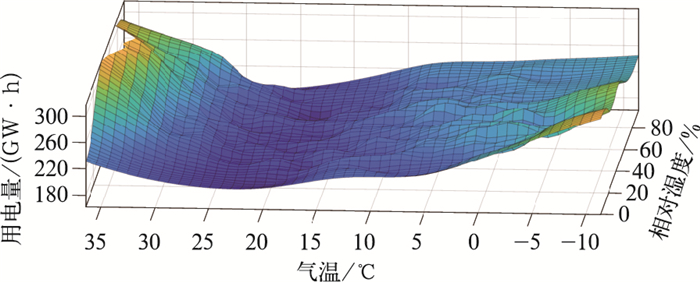图 1 2014—2018年天津日用电量与气温和相对湿度之间的三维关系分布 Fig. 1 Distribution of three-dimensional relationship between daily electricity load and temperature and relative humidity in Tianjin during 2014-2018
1.2 日用电量预测模型

Scapin et al (2016)的研究结果表明，用电量预测模型主要受周期性变化和气象条件变化两部分影响，而用电量随气象条件的变化表现为线性和非线性特征，Brown et al(2016)认为线性不对称模型在评估多种气象条件对用电量的影响及人为控制变量方面存在明显优势，故文中以此模型来确定分段阈值。具体公式如下所示：

 $D=\sum\limits_{i=0}^{m} \alpha_{i} t^{i}+\sum\limits_{j=1}^{4} \beta_{j} I_{j}+\sum\limits_{k=1}^{n} \gamma_{k} V_{k}+\varepsilon$ (1)

 $D F=\sum\limits_{k=1}^{n} \gamma_{k} V_{k}+\varepsilon$ (2)
1.3 评估模型

Hansen(1999)在求取分段阈值的研究中以残差平方和的最小化为条件来确定阈值，本文采用类似的均方根误差(RMSE)的最小化来选取最优气温阈值，该模型也被广泛用于评估各类模型预测效果(吴琼等, 2020;任永建等, 2020;张天航等, 2020)，具体计算公式为：

 $R M S E=\sqrt{\frac{1}{n} \sum\limits_{i=1}^{n}\left(y_{i}-\hat{y}_{i}\right)^{2}}$ (3)

 $M A P E=\frac{100 \%}{n} \sum\limits_{i=1}^{n}\left|\frac{y_{i}-\widehat{y}_{i}}{y_{i}}\right|$ (4)

2 基于温湿度的日用电量分段的计算方法

 $D_{j}=a T_{j}{ }^{2}+b T_{j}+c$ (5)

 $k_{j}=\frac{\Delta D}{\Delta T}=\frac{D_{j}-D_{j-1}}{T_{j}-T_{j-1}}$ (6)

 $D_{m}<\frac{1}{3} \text { & } D_{n}<\frac{1}{3}$ (7)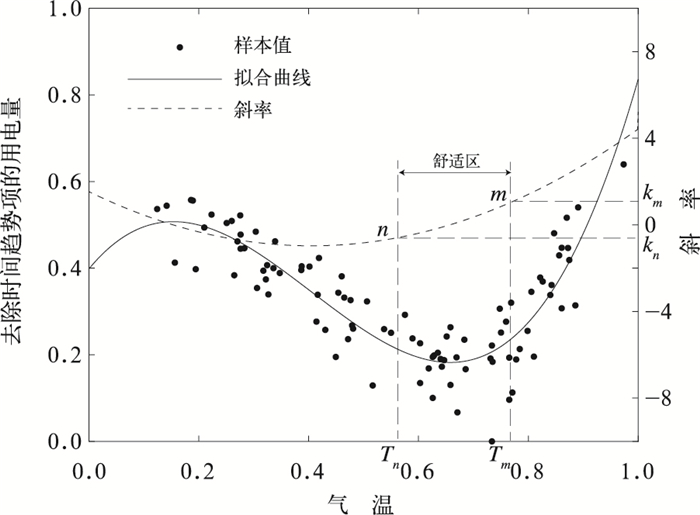图 2 相对湿度为55%~60%时气温和用电量归一化数据的非线性拟合及分段示意图 Fig. 2 Nonlinear fitting and segmental diagram of normalized temperature and electricity load in the range of 55%-60% relative humidity

 $T_{m, k_{m}} =\omega_{m, k_{m}} R H_{m, k_{m}}+\theta_{m, k_{m}}$ (8)
 $T_{n, k_{n}} =\omega_{n, k_{n}} R H_{n, k_{n}}+\theta_{n, k_{n}}$ (9)

 $Q_{m, k_{m}}=\sqrt{\sum\limits_{i=1}^{8}\left(T_{m, k_{m}, i}-\omega_{m, k_{m}} R H_{m, k_{m}, i}-\theta_{m, k_{m}}\right)^{2}}$ (10)
 $Q_{n, k_{n}}=\sqrt{\sum\limits_{i=1}^{8}\left(T_{n, k_{n}, i}-\omega_{n, k_{n}} R H_{n, k_{n}, i}-\theta_{n, k_{n}}\right)^{2}}$ (11)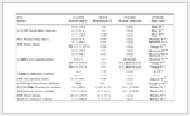表 1 km=1.0, kn=0.8时，不同相对湿度范围内的用电量分段的气温阈值 Table 1 Temperature thresholds for electricity load segments during different relative humidity when km=1.0, kn=0.8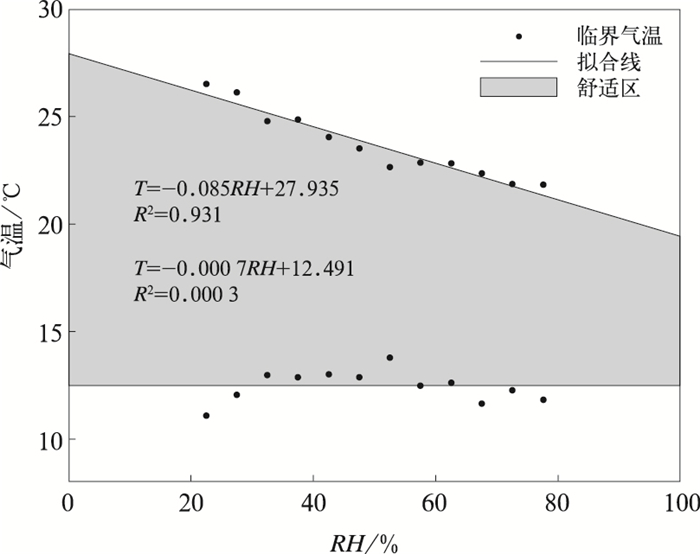图 3 km=1.0, kn=0.8时，用电量分段的温湿度拟合曲线 Fig. 3 Fitting curve of temperature and RH by different electricity load segments when km=1.0, kn=0.8
3 基于温湿度的日用电量分段模型与预测效果检验 3.1 基于温湿度的日用电量分段模型表 2 基于温湿度的日用电量分段模型——以天津为例 Table 2 Segmental model of daily electricity load based on temperature and humidity in Tianjin
3.2 预测效果检验

 $D F= \begin{cases}\mu_{1}+\omega_{1} T+\varepsilon & TT \geqslant n \\ \mu_{3}+\omega_{3} T+\varepsilon & T \geqslant m\end{cases}$ (12)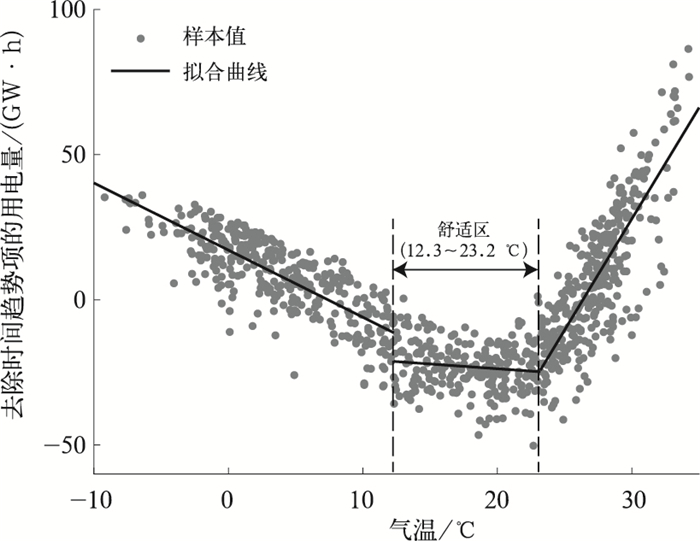图 4 基于TR模型的天津地区气温和用电量的拟合分布曲线 Fig. 4 Fitting curve of temperature and electricity load in Tianjin based on threshold regression model

3.2.1 线性模型下的预测效果检验表 3 基于公式(1)的各类分段方法下日用电量预测效果对比 Table 3 Comparison of daily electricity load forecasting result by different segmentation methods based on Eq.(1)

3.2.2 非线性模型下的预测效果检验

 $D=\sum\limits_{i=0}^{m} \alpha_{i} t^{i}+\sum\limits_{j=1}^{4} \beta_{j} I_{j}+\sum\limits_{k=1}^{n} \gamma_{k} V_{k}+\sum\limits_{g=1}^{2} \omega_{g} L_{g} D_{t-1}+\varepsilon$ (13)

 $\begin{gathered} T P=\left(T-\bar{T}_{m}\right) \theta\left(T>\bar{T}_{m}\right)+\left(\bar{T}_{n}-T\right) \times \\ \theta\left(\bar{T}_{n}>T\right)+T \theta\left(\bar{T}_{m} \geqslant T \geqslant \bar{T}_{n}\right) \end{gathered}$ (14)表 4 基于式(12)各类分段方法下日用电量预测效果对比 Table 4 Comparison of daily electricity load forecasting result by different segmentation methods based on Eq.(12)

4 结论与讨论

(1) 日用电量对气温和相对湿度均表现出高敏感性，随着相对湿度的变化，用电量和气温之间的低敏感区范围(即舒适区)会发生明显偏移，并在冷却区与舒适区之间表现更为显著。

(2) 分析舒适区的气温阈值发现，随着相对湿度增大，Tm向低温一侧偏移，Tn则变化不大，因此舒适区范围会随相对湿度增大而变窄。

(3) 对预测效果评估结果显示，线性模型中，预测效果较传统“V”型和“U”型均有所提升，针对舒适区和冷却区过渡区，RMSE和MAPE值分别减小了0.759 GW·h和0.215%；非线性模型中同样表现出了较好的提升效果，RMSE和MAPE值分别减小了0.647 GW·h和0.209%，不同模型的预测效果均有提升且效果稳定，表明新方法在提升对气象敏感的电力需求预测的准确性上具有显著意义。